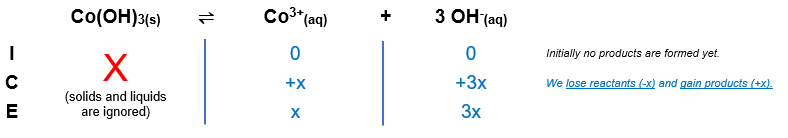# Problem: Calculate the molar solubility of Co(OH)3, Ksp = 2.5 X 10 -43.

🤓 Based on our data, we think this question is relevant for Professor Jackman's class at UT.

###### FREE Expert Solution

Co(OH)3(s)  Co3+(aq) + 3 OH(aq)The Ksp expression for Co(OH)3 is:

$\overline{){{\mathbf{K}}}_{{\mathbf{sp}}}{\mathbf{=}}\frac{\mathbf{products}}{\overline{)\mathbf{reactants}}}{\mathbf{=}}{\mathbf{\left[}}{{\mathbf{Co}}}^{\mathbf{3}\mathbf{+}}{\mathbf{\right]}}{\mathbf{\left[}}{{\mathbf{OH}}}^{{\mathbf{-}}}{{\mathbf{\right]}}}^{{\mathbf{3}}}}$

${\mathbf{K}}_{\mathbf{sp}}\mathbf{=}\mathbf{\left[}{\mathbf{Co}}^{\mathbf{3}\mathbf{+}}\mathbf{\right]}\mathbf{\left[}{\mathbf{OH}}^{\mathbf{-}}{\mathbf{\right]}}^{\mathbf{3}}$###### Problem Details

Calculate the molar solubility of Co(OH)3, Ksp = 2.5 X 10 -43.